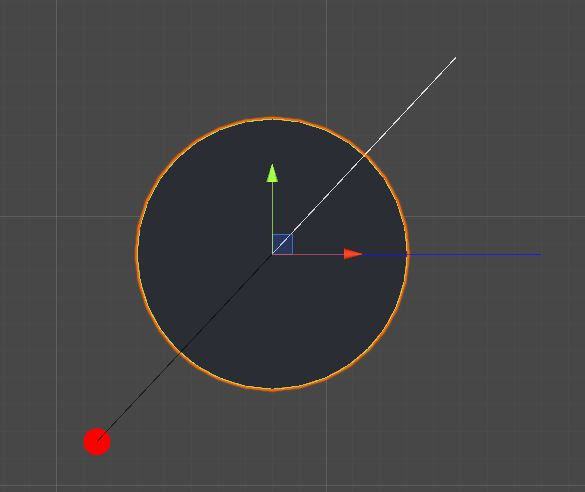# Calculate launch angle based on launch direction

I’m working with a setup like this:Where the vectors all start at transform.position and end as follows:

• Black: transform.position + HandlePosition
• Blue: transform.position + Vector2.right
• White: transform.position - HandlePosition.normalized

The idea is that i can move the red handle around to change the White vector (the launch direction of the object).

Now what I would like to get is the angle between the White and Blue vectors.

I want to get this angle so that I can calculate the trajectory of the object and display a trajectory line.

Edit:
Given the example shown on the picture, I would expect an angle of about 45 degrees.

Check out Mathf.Atan2

The solution was to calculate a new vector based on the White vector and then use it in the Mathf.Atan2 method.

``````Vector3 newVector = transform.position - White;
float angle = Mathf.Atan2(newVector.y, newVector.x) *Mathf.Rad2Deg;
``````

This however gives values ranging from -180 to 180, to convert this to a 0 - 360 degree value I simply added 180 to the result of previous calculation.

``````angle+= 180;
``````

This works nicely for what I’m trying to do.

Thanks to @Pangamini for guiding me in the right direction.